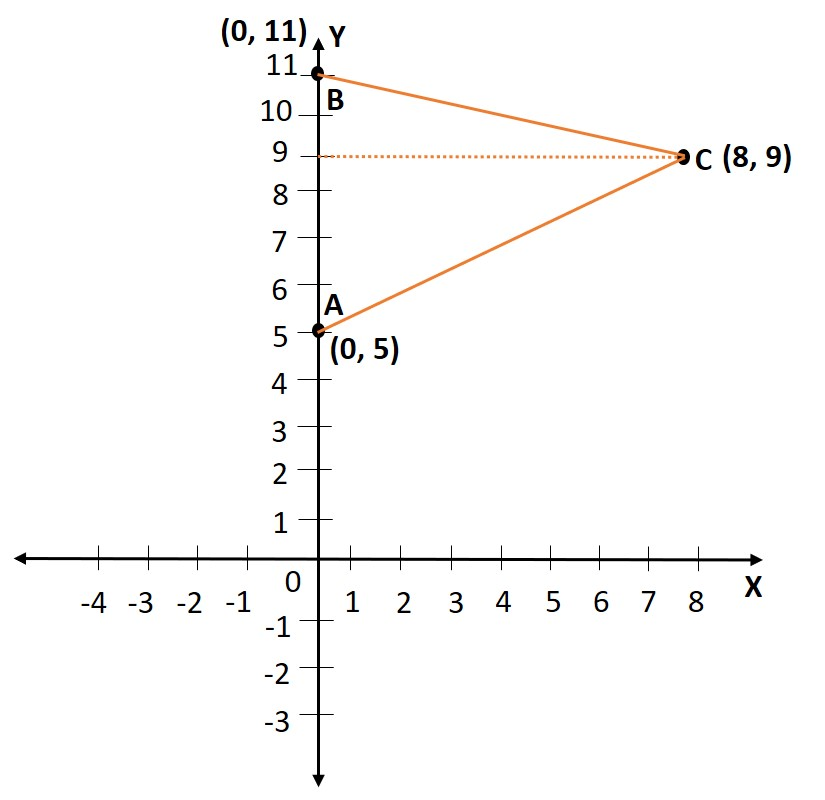# Extra Questions Class 9 Maths | Coordinate Geometry Q4

###### NCERT Chapter 3 | Area of a triangle | Cartesian PlaneClass 9 Maths Questions

Question 4: What is the area of the triangle formed by joining points (0, 5), (0, 11) and (8, 9)?

## NCERT Solution to Class 9 Maths

### Explanatory Answer | Chapter 3 Extra Question 4Let the coordinates of the vertices of the triangle A, B, and C be (0, 5), (0, 11), and (8, 9) respectively.
Points A and B lie on the y – axis because their abscissa (x- coordinate) are zero.

Let AB be the base of the triangle.
Draw CD Parallel to x – axis. Coordinates of point D are (0, 9).
Because CD is perpendicular to AB, and is drawn from the vertex opposite to AB, CD is the altitude to base AB.

Length of AB = 6 Units.
Length of CD = 8 units.

Area of the triangle = $\frac{1}{2}$ x base x altitude
= $\frac{1}{2}$ x 6 x 8 = 24 sq. units

###### Free CBSE Online CoachingClass 9 Maths

Register in 2 easy steps and
Start learning in 5 minutes!